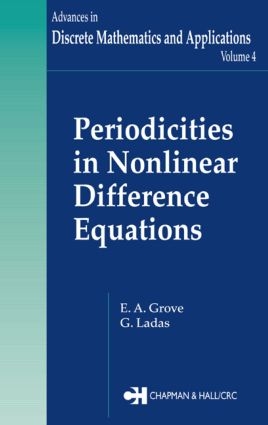Periodicities in Nonlinear Difference Equations

1st Edition

Chapman and Hall/CRC

392 pages | 50 B/W Illus.

Purchasing Options:\$ = USD
Hardback: 9780849331565
pub: 2004-12-16
SAVE ~\$35.00
\$175.00
\$140.00
x
eBook (VitalSource) : 9780429128202
pub: 2004-12-16
from \$28.98

FREE Standard Shipping!

Description

Sharkovsky's Theorem, Li and Yorke's "period three implies chaos" result, and the (3x+1) conjecture are beautiful and deep results that demonstrate the rich periodic character of first-order, nonlinear difference equations. To date, however, we still know surprisingly little about higher-order nonlinear difference equations.

During the last ten years, the authors of this book have been fascinated with discovering periodicities in equations of higher order which for certain values of their parameters have one of the following characteristics:

1. Every solution of the equation is periodic with the same period.

2. Every solution of the equation is eventually periodic with a prescribed period.

3. Every solution of the equation converges to a periodic solution with the same period.

This monograph presents their findings along with some thought-provoking questions and many open problems and conjectures worthy of investigation. The authors also propose investigation of the global character of solutions of these equations for other values of their parameters and working toward a more complete picture of the global behavior of their solutions.

With the results and discussions it presents, Periodicities in Nonlinear Difference Equations places a few more stones in the foundation of the basic theory of nonlinear difference equations. Researchers and graduate students working in difference equations and discrete dynamical systems will find much to intrigue them and inspire further work in this area.

Reviews

"The advantage of the book is not only the presentation of new results, but also the formulation of many open problems and conjectures which shall stimulate further investigations of researchers and graduate students."

- Lothar Berg, Zentralblatt MATH, 2006

PRELIMINARIES

EQUATIONS WITH PERIODIC SOLUTIONS

EQUATIONS WITH EVENTUALLY PERIODIC SOLUTIONS

CONVERGENCE TO PERIODIC SOLUTIONS

THE EQUATION x(n+1) = a + gx(n¡-(2k+1) )+ d(xn-2l )/ A + x(n-2l)

MAX EQUATIONS WITH PERIODIC SOLUTIONS

MAX EQUATIONS WITH PERIODIC COEFFICIENTS

EQUATIONS IN THE SPIRIT OF THE (3x+1) CONJECTURE

Bibliography

References

Index